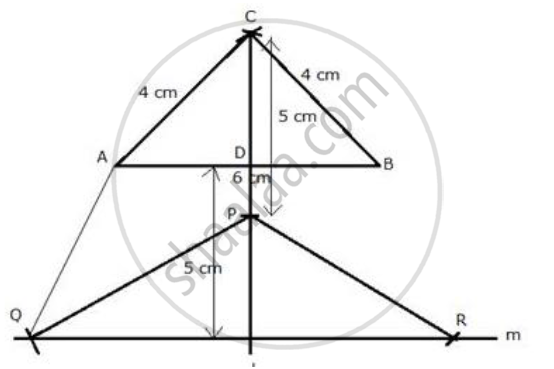# Construct an Isosceles Triangle Abc Such that Ab = 6cm, Bc = Ac = 4cm. Bisect ∠C Internally and Mark a Point P on this Bisector Such that Cp = 5 Cm. Find the Points Q and R Which a - Mathematics

Sum

Construct an isosceles triangle ABC such that AB = 6cm, BC = AC = 4cm. Bisect ∠C internally and mark a point P on this bisector such that CP = 5 cm. Find the points Q and R which are 5 cm from P and also 5 cm from the line AB.

#### SolutionSteps of Construction:
i) Draw a line segment AB = 6 cm.
ii) With centers A and B and radius 4 cm, draw two arcs which intersect each other at C.
iii) Join CA and CB.
iv) Draw the angle bisector of angle C and cut off CP = 5 cm.
v) A line m is drawn parallel to AB at a distance of 5 cm.
vi) P as centre and radius 5 cm, draw arcs which intersect the line m at Q and R.
vii) Join PQ, PR and AQ.
Q and R are the required points.

Concept: Constructions Under Loci
Is there an error in this question or solution?

#### APPEARS IN

Selina Concise Maths Class 10 ICSE
Chapter 16 Loci (Locus and Its Constructions)
Exercise 16 (B) | Q 28 | Page 241